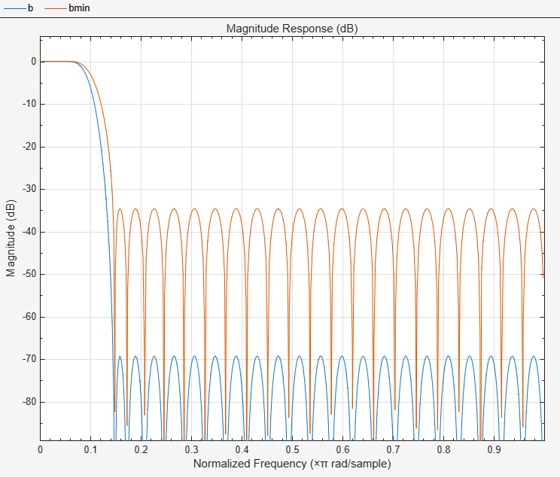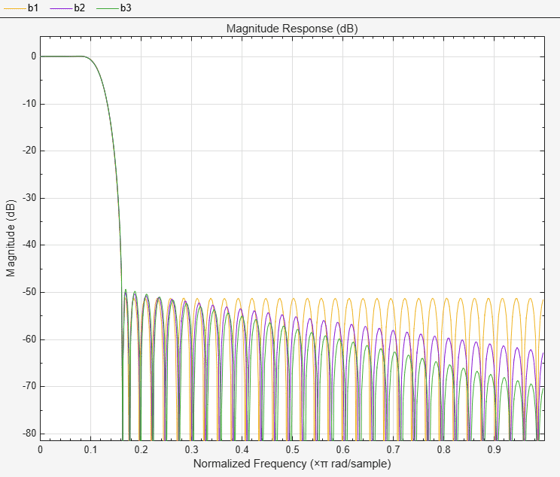# firnyquist

Lowpass Nyquist (Lth-band) FIR filter

## Syntax

```b = firnyquist(n,l,r) b = firnyquist('minorder',l,r,dev) b = firnyquist(n,l,r,decay) b = firnyquist(n,l,r,'nonnegative') b = firnyquist(n,l,r,'minphase') ```

## Description

`b = firnyquist(n,l,r)` designs an Nth order, Lth band, Nyquist FIR filter with a rolloff factor `r` and an equiripple characteristic.

The rolloff factor `r` is related to the normalized transition width `tw` by tw = 2π(r/l) (rad/sample). The order, `n`, must be even. `l` must be an integer greater than one. If `l` is not specified, it defaults to `4`. `r` must satisfy `0< r < 1`. If `r` is not specified, it defaults to `0.5`.

`b = firnyquist('minorder',l,r,dev)` designs a minimum-order, Lth band Nyquist FIR filter with a rolloff factor `r` using the Kaiser window. The peak ripple is constrained by the scalar `dev`.

`b = firnyquist(n,l,r,decay)` designs an Nth order (`n`), Lth band (`l`), Nyquist FIR filter where the scalar `decay`, specifies the rate of decay in the stopband. `decay` must be nonnegative. If you omit or leave it empty, `decay` defaults to `0` which yields an equiripple stopband. `A` nonequiripple stopband (`decay` ≠0) may be desirable for decimation purposes.

`b = firnyquist(n,l,r,'nonnegative')` returns an FIR filter with nonnegative zero-phase response. This filter can be spectrally factored into minimum-phase and maximum-phase “square-root” filters. This allows you to use the spectral factors in applications such as matched-filtering.

`b = firnyquist(n,l,r,'minphase')` returns the minimum-phase spectral factor `bmin` of order `n`. `bmin` meets the condition `b=``conv``(bmin,bmax) `so that `b` is an Lth band FIR Nyquist filter of order 2n with filter rolloff factor `r`. Obtain `bmax`, the maximum phase spectral factor by reversing the coefficients of `bmin`. For example, `bmax = bmin(end:-1:1)`.

## Examples

collapse all

This example designs a minimum phase factor of a Nyquist filter.

```bmin = firnyquist(47,10,.45,'minphase'); b = firnyquist(2*47,10,.45,'nonnegative'); [h,w,s] = freqz(b); hmin = freqz(bmin); fvtool(b,1,bmin,1);```This example compares filters with different decay rates.

```b1 = firnyquist(72,8,.3,0); % Equiripple b2 = firnyquist(72,8,.3,15); b3 = firnyquist(72,8,.3,25); fvtool(b1,1,b2,1,b3,1);```## References

T. Saramaki, Finite Impulse Response Filter Design, Handbook for Digital Signal Processing, Mitra, S.K. and J.F. Kaiser Eds. Wiley-Interscience, N.Y., 1993, Chapter 4.